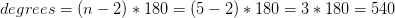## Example Questions

### Example Question #3 : Pentagons

Find the sum of all the angles in a pentagon.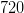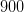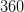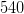Explanation:

To solve, simply use the formula to find the total degrees in a polygon, where n is thenumber of vertices.

In this particular case, a pentagon is a shape that has five sides and thus has five vertices.

Thus,